Скачать презентацию A Working Knowledge of Computational Complexity for an

f4d6ef6c178d778a2110bee104c3e434.ppt

• Количество слайдов: 55
A Working Knowledge of Computational Complexity for an Optimizer ORF 363/COS 323 Instructor: Amir Ali Ahmadi TAs: B. El Khadir, G. Hall, X. Li, K. Wang, J. Ye, J. Zhang Fall 2016 1
Why computational complexity? §What is computational complexity theory? It’s a branch of mathematics that provides a formal framework for studying how efficiently one can solve problems on a computer. §This is absolutely crucial to optimization and many other computational sciences. §In optimization, we are constantly looking for algorithms to solve various problems as fast as possible. So it is of immediate interest to understand the fundamental limitations of efficient algorithms. §So far in this class we’ve had a rule of thumb for checking if an optimization problem is “easy”: §See if it’s convex! §But this only scratches the surface. Are all nonconvex problems hard? Are some of them hard? Are there even convex problems that are hard? § What does it even mean to be hard? ! §Let’s begin by understanding what it means to have a “problem”! 2
Optimization problems/Decision problems/Search problems Optimization problem: Decision problem: (answer to a decision question is just YES or NO) Search problem: §It turns out that all three problems are equivalent, in the sense that if you could solve one efficiently, you could also solve the other two. See Ex. 8. 1, 8. 2 of [DPV]. §We will focus on decision problems, since it’s a bit cleaner to develop theory there. 3
A “problem” versus a “problem instance” §A (decision) problem is a general description of a problem to be answered with yes or no. §Every decision problem has a finite input that needs to be specified for us to choose a yes/no answer. §Each such input defines an instance of the problem. §A decision problem has an infinite number of instances. (Why doesn’t it make sense to study problems with a finite number of instances? ) §Different instances of the STABLE SET problem: (It is common to use capital letters for the name of a decision problem. ) 4
Examples of decision problems §LINEQ An instance of LINEQ: §ZOLINEQ An instance of ZOLINEQ: §Remark. Input is rational so we can represent it with a finite number of bits. This is the so-called “bit model of computation ”, aka the “Turing model. ’’ 5
Examples of decision problems §LP (This is equivalent to testing LP feasibility (why? ). ) An instance of LP: §IP 6
Examples of decision problems Let’s look at a problem we have seen… An instance of MAXFLOW: Can you formulate the decision problem? §MAXFLOW 7
Examples of decision problems §For example, the following graph is 3 -colorable. §Graph coloring has important applications in job scheduling. §COLORING §We want to understand how fast can all these problems be solved? 8
Size of an instance §To talk about the running time of an algorithm, we need to have a notion of the “size of the input”. §Of course, an algorithm is allowed to take longer on larger instances. §COLORING §STABLE SET §Reasonable candidates for input size: §Number of nodes n §Number of nodes + number of edges (number of edges can at most be n(n-1)/2) §Number of bits required to store the adjacency matrix of the graph 9
Size of an instance §In general, can think of input size as the total number of bits required to represent the input. §For example, consider our LP problem: §LP 10
Useful notation for referring to running times 11
Polynomial-time and exponential-time algorithms Something you all know: Poly-time: Exp-time: 12
See page 233 of [DPV] for the story. Sissa (credited for creating the game of chess) 13
Comparison of running times Image credit: [GJ 79] 14
Can Moore’s law come to rescue? Image credit: [GJ 79] 15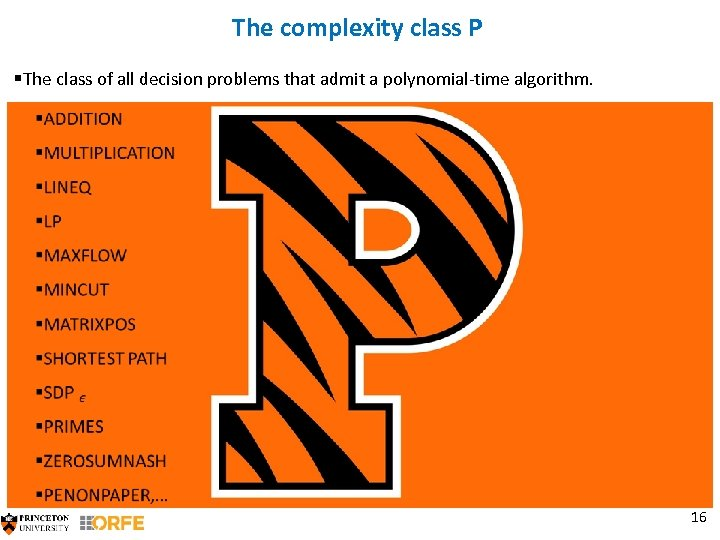The complexity class P §The class of all decision problems that admit a polynomial-time algorithm. 16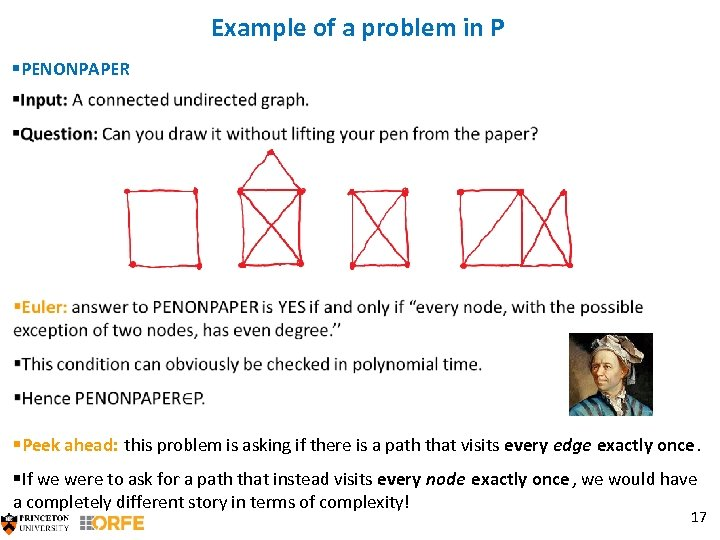Example of a problem in P §PENONPAPER §Peek ahead: this problem is asking if there is a path that visits every edge exactly once. §If we were to ask for a path that instead visits every node exactly once , we would have a completely different story in terms of complexity! 17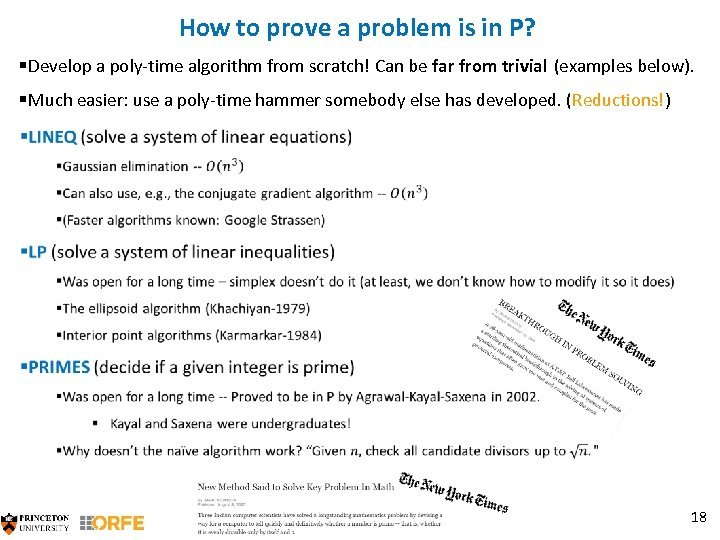How to prove a problem is in P? §Develop a poly-time algorithm from scratch! Can be far from trivial (examples below). §Much easier: use a poly-time hammer somebody else has developed. (Reductions!) 18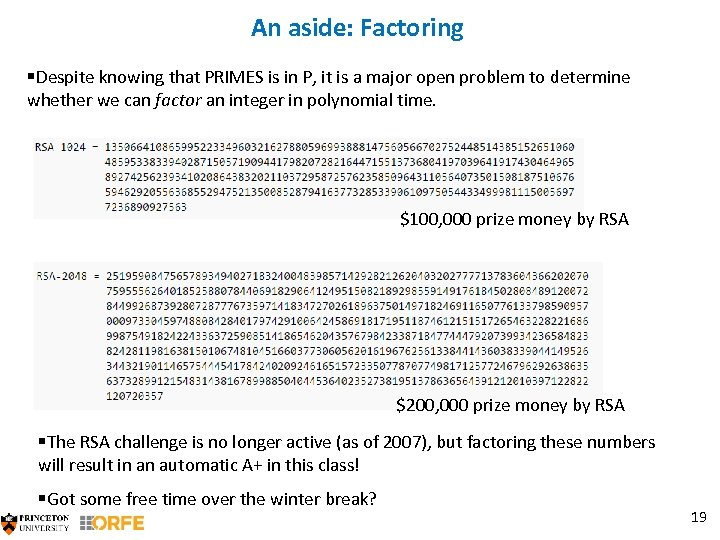An aside: Factoring §Despite knowing that PRIMES is in P, it is a major open problem to determine whether we can factor an integer in polynomial time. \$100, 000 prize money by RSA \$200, 000 prize money by RSA §The RSA challenge is no longer active (as of 2007), but factoring these numbers will result in an automatic A+ in this class! §Got some free time over the winter break? 19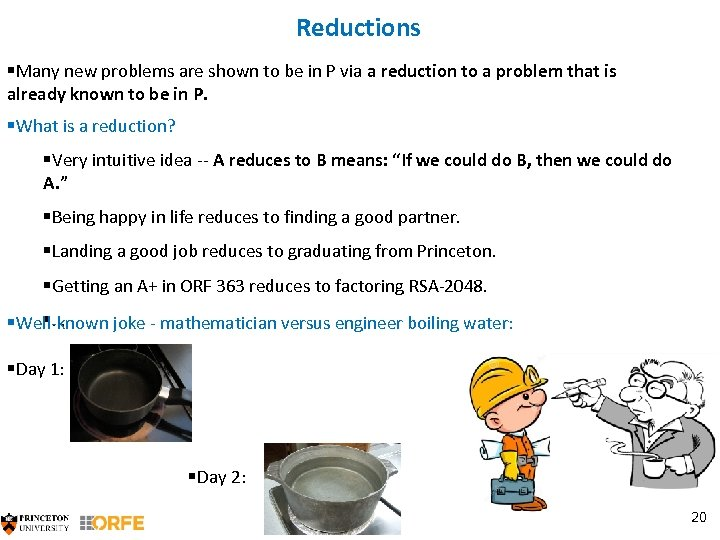Reductions §Many new problems are shown to be in P via a reduction to a problem that is already known to be in P. §What is a reduction? §Very intuitive idea -- A reduces to B means: “If we could do B, then we could do A. ” §Being happy in life reduces to finding a good partner. §Landing a good job reduces to graduating from Princeton. §Getting an A+ in ORF 363 reduces to factoring RSA-2048. §… §Well-known joke - mathematician versus engineer boiling water: §Day 1: §Day 2: 20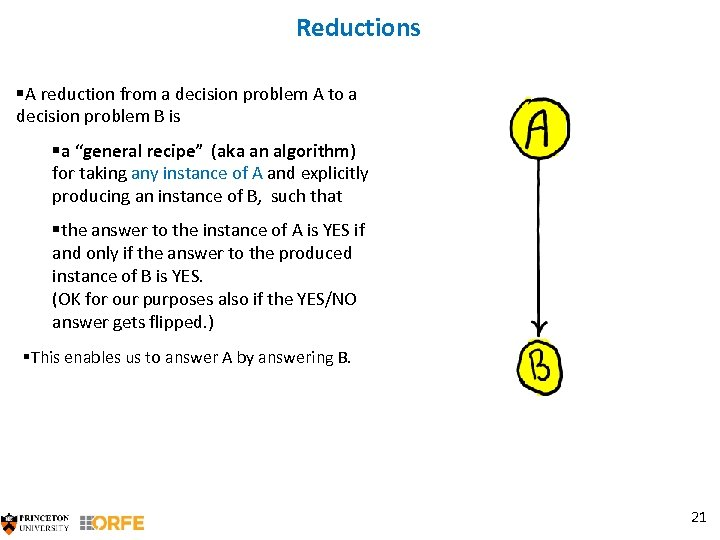Reductions §A reduction from a decision problem A to a decision problem B is §a “general recipe” (aka an algorithm) for taking any instance of A and explicitly producing an instance of B, such that §the answer to the instance of A is YES if and only if the answer to the produced instance of B is YES. (OK for our purposes also if the YES/NO answer gets flipped. ) §This enables us to answer A by answering B. 21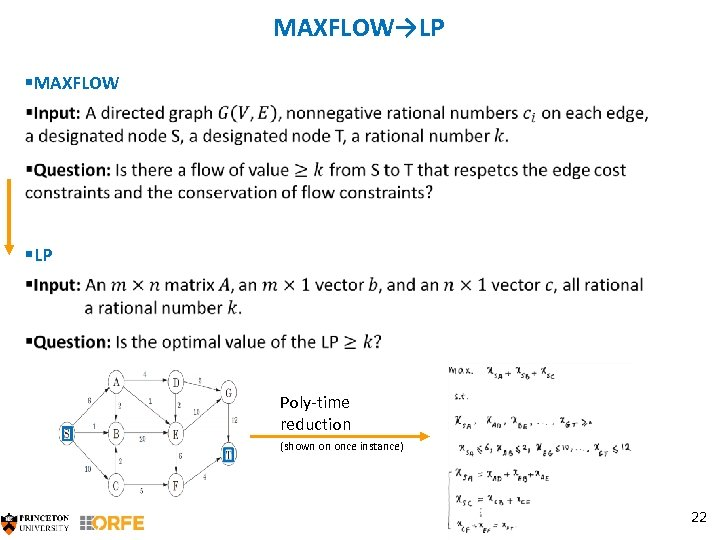MAXFLOW→LP §MAXFLOW §LP Poly-time reduction (shown on once instance) 22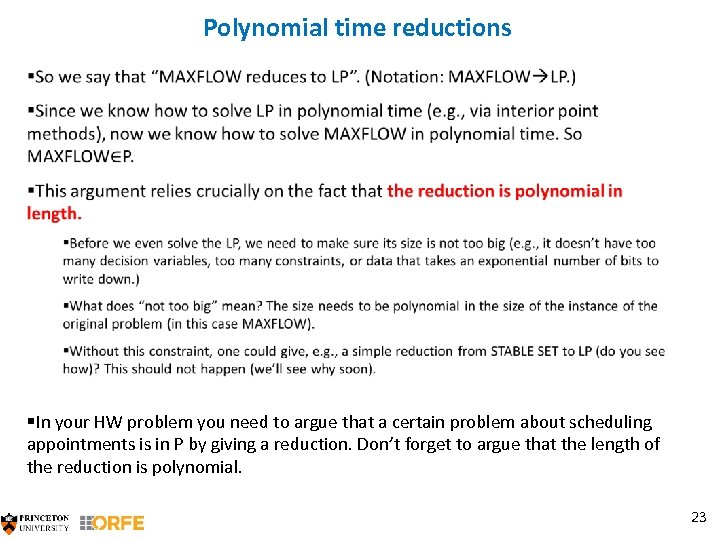Polynomial time reductions §In your HW problem you need to argue that a certain problem about scheduling appointments is in P by giving a reduction. Don’t forget to argue that the length of the reduction is polynomial. 23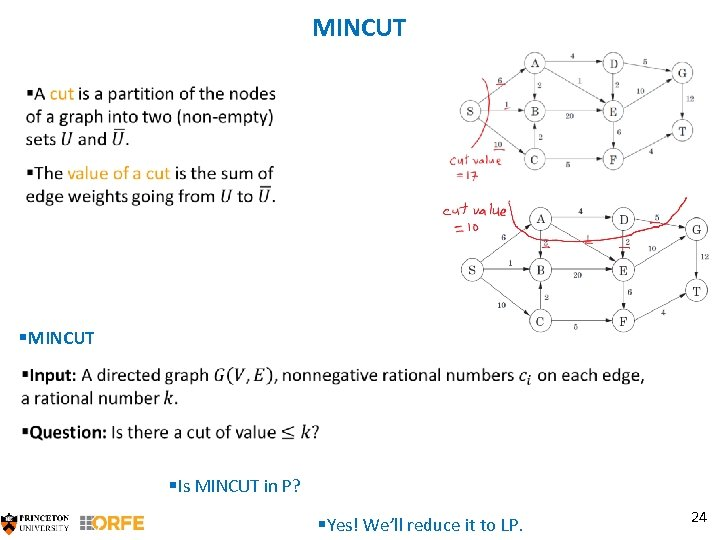MINCUT §Is MINCUT in P? §Yes! We’ll reduce it to LP. 24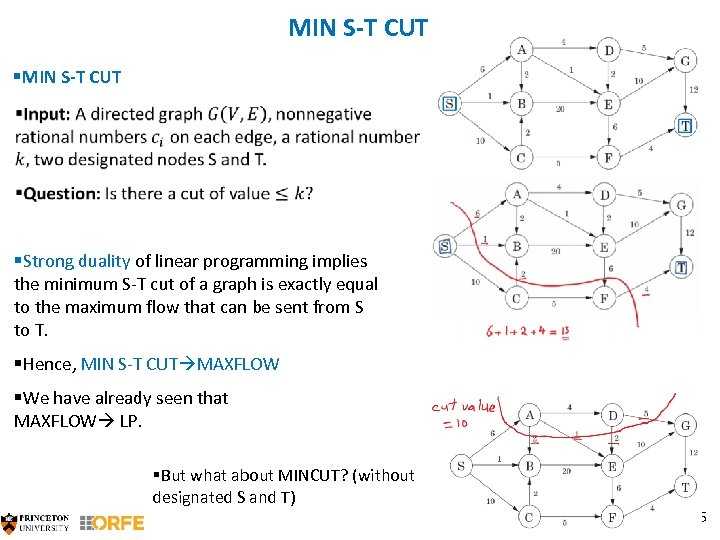MIN S-T CUT §MIN S-T CUT §Strong duality of linear programming implies the minimum S-T cut of a graph is exactly equal to the maximum flow that can be sent from S to T. §Hence, MIN S-T CUT MAXFLOW §We have already seen that MAXFLOW LP. §But what about MINCUT? (without designated S and T) 25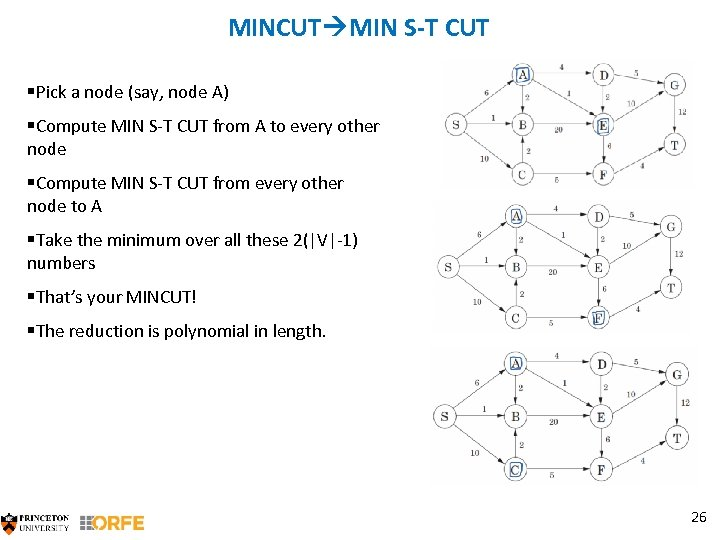MINCUT MIN S-T CUT §Pick a node (say, node A) §Compute MIN S-T CUT from A to every other node §Compute MIN S-T CUT from every other node to A §Take the minimum over all these 2(|V|-1) numbers §That’s your MINCUT! §The reduction is polynomial in length. 26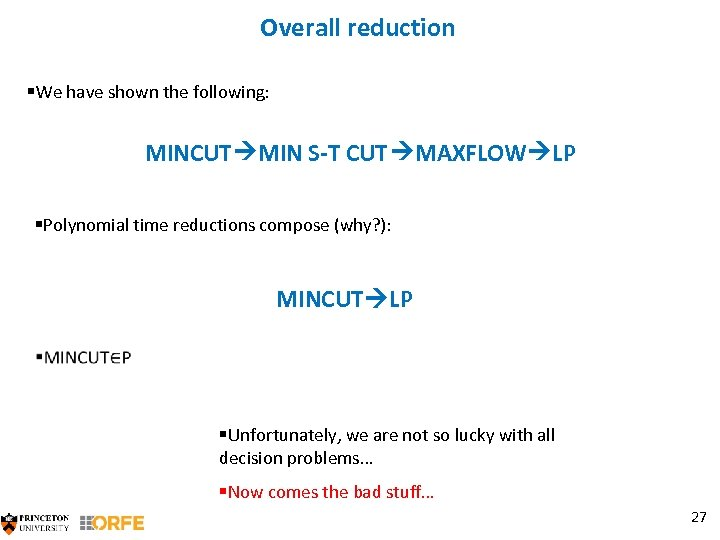Overall reduction §We have shown the following: MINCUT MIN S-T CUT MAXFLOW LP §Polynomial time reductions compose (why? ): MINCUT LP §Unfortunately, we are not so lucky with all decision problems… §Now comes the bad stuff… 27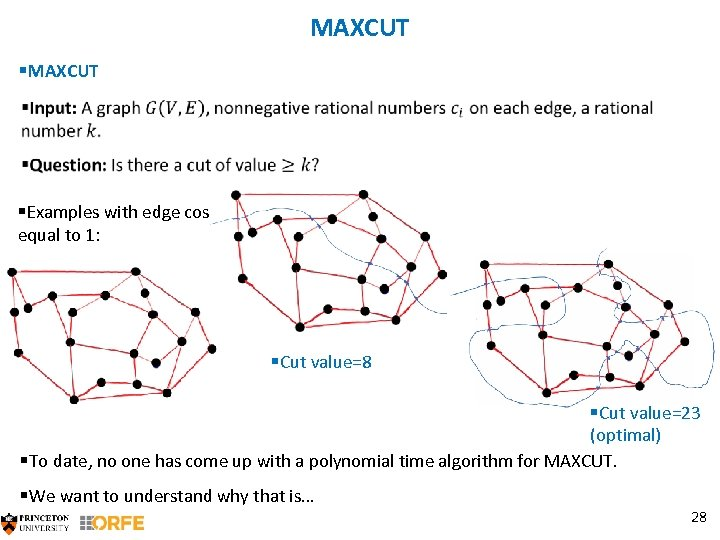MAXCUT §MAXCUT §Examples with edge costs equal to 1: §Cut value=8 §Cut value=23 (optimal) §To date, no one has come up with a polynomial time algorithm for MAXCUT. §We want to understand why that is… 28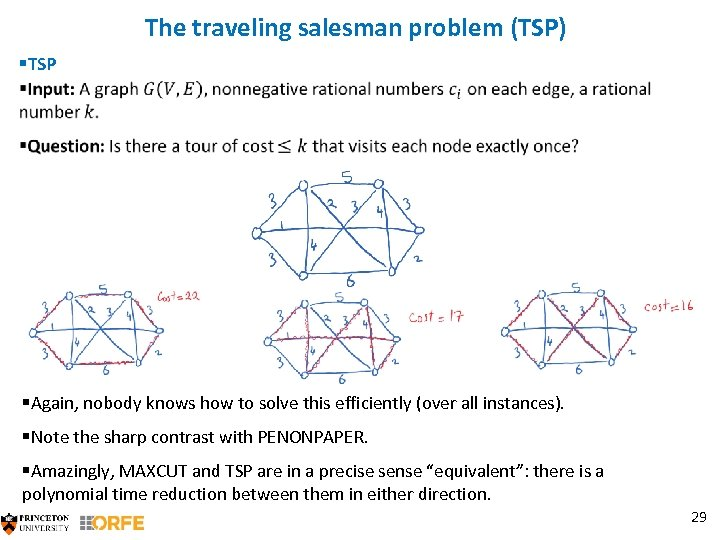The traveling salesman problem (TSP) §TSP §Again, nobody knows how to solve this efficiently (over all instances). §Note the sharp contrast with PENONPAPER. §Amazingly, MAXCUT and TSP are in a precise sense “equivalent”: there is a polynomial time reduction between them in either direction. 29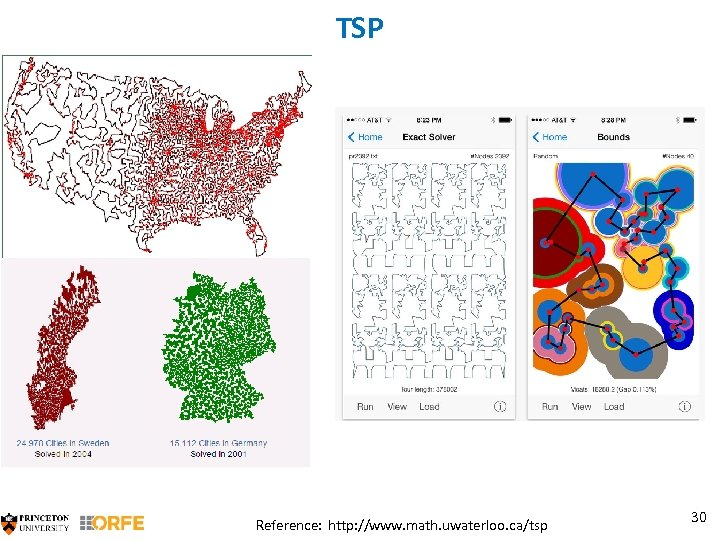TSP Reference: http: //www. math. uwaterloo. ca/tsp 30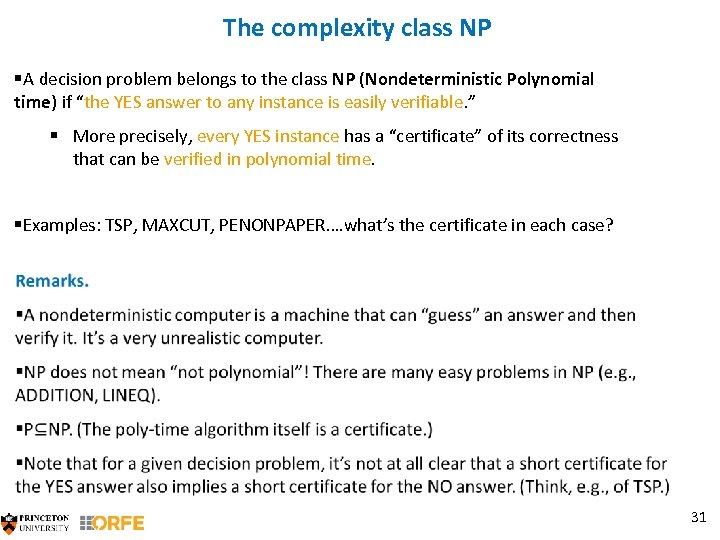The complexity class NP §A decision problem belongs to the class NP (Nondeterministic Polynomial time) if “the YES answer to any instance is easily verifiable. ” § More precisely, every YES instance has a “certificate” of its correctness that can be verified in polynomial time. §Examples: TSP, MAXCUT, PENONPAPER…. what’s the certificate in each case? 31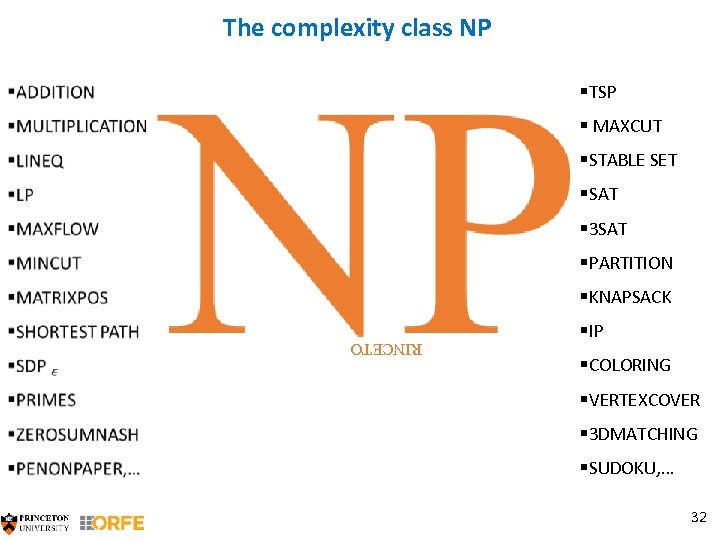The complexity class NP §TSP § MAXCUT §STABLE SET §SAT § 3 SAT §PARTITION §KNAPSACK §IP §COLORING §VERTEXCOVER § 3 DMATCHING §SUDOKU, … 32 RINCETO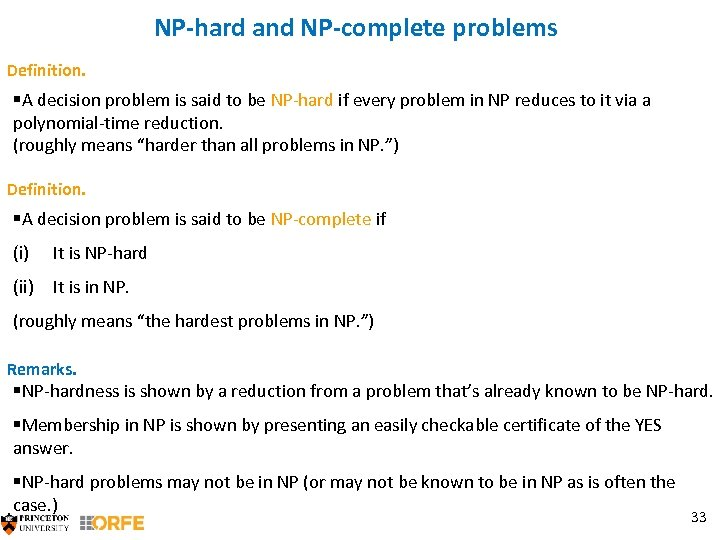NP-hard and NP-complete problems Definition. §A decision problem is said to be NP-hard if every problem in NP reduces to it via a polynomial-time reduction. (roughly means “harder than all problems in NP. ”) Definition. §A decision problem is said to be NP-complete if (i) It is NP-hard (ii) It is in NP. (roughly means “the hardest problems in NP. ”) Remarks. §NP-hardness is shown by a reduction from a problem that’s already known to be NP-hard. §Membership in NP is shown by presenting an easily checkable certificate of the YES answer. §NP-hard problems may not be in NP (or may not be known to be in NP as is often the case. ) 33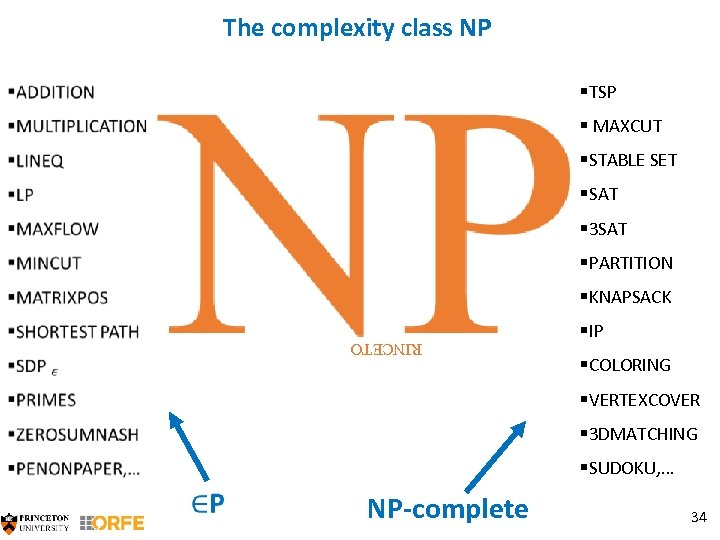The complexity class NP §TSP § MAXCUT §STABLE SET §SAT § 3 SAT §PARTITION §KNAPSACK §IP §COLORING RINCETO §VERTEXCOVER § 3 DMATCHING §SUDOKU, … NP-complete 34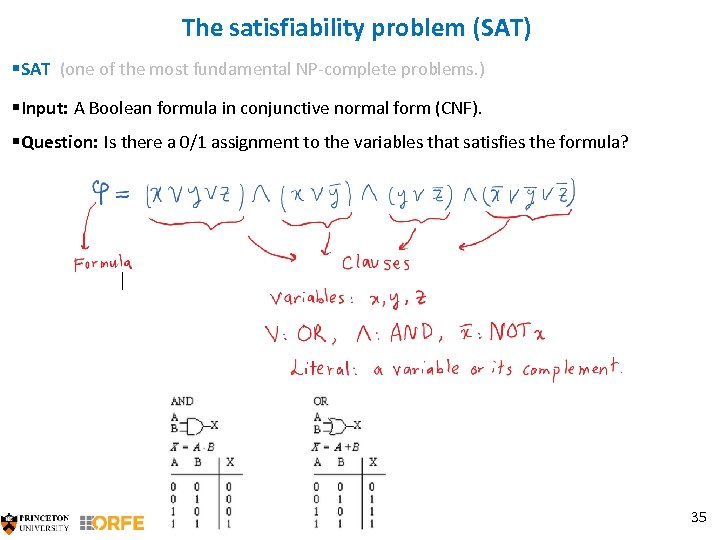The satisfiability problem (SAT) §SAT (one of the most fundamental NP-complete problems. ) §Input: A Boolean formula in conjunctive normal form (CNF). §Question: Is there a 0/1 assignment to the variables that satisfies the formula? 35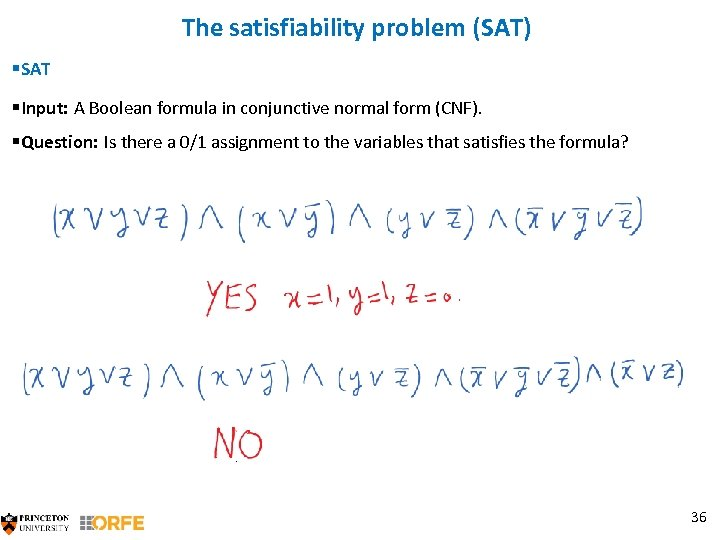The satisfiability problem (SAT) §SAT §Input: A Boolean formula in conjunctive normal form (CNF). §Question: Is there a 0/1 assignment to the variables that satisfies the formula? 36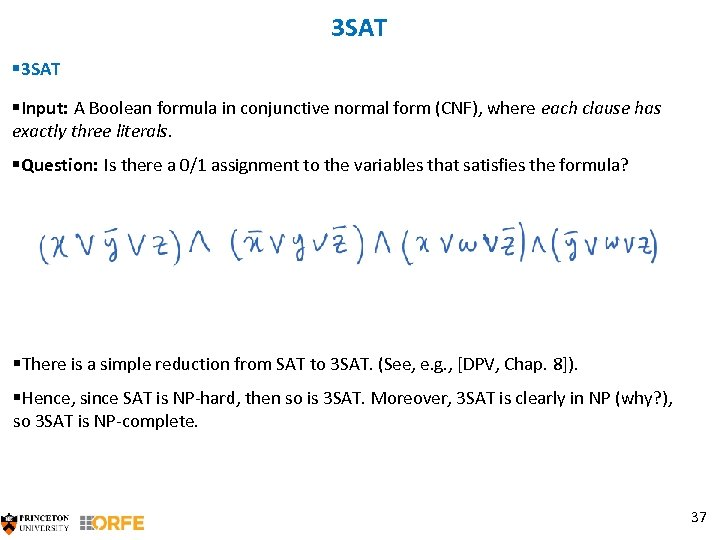3 SAT §Input: A Boolean formula in conjunctive normal form (CNF), where each clause has exactly three literals. §Question: Is there a 0/1 assignment to the variables that satisfies the formula? §There is a simple reduction from SAT to 3 SAT. (See, e. g. , [DPV, Chap. 8]). §Hence, since SAT is NP-hard, then so is 3 SAT. Moreover, 3 SAT is clearly in NP (why? ), so 3 SAT is NP-complete. 37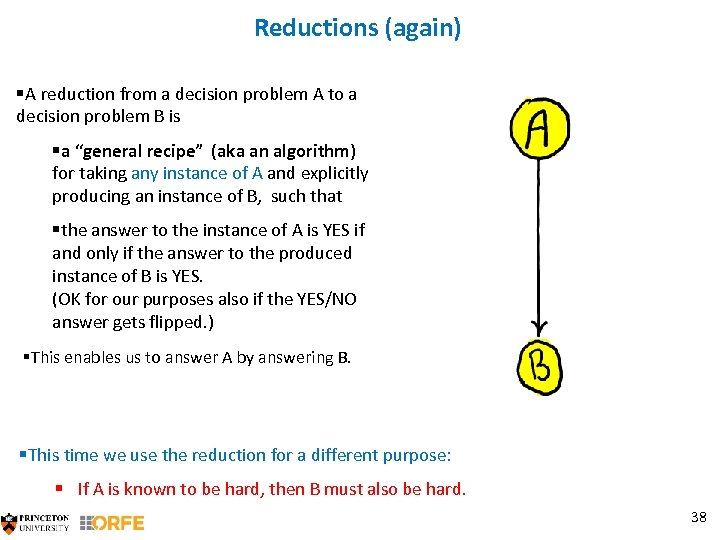Reductions (again) §A reduction from a decision problem A to a decision problem B is §a “general recipe” (aka an algorithm) for taking any instance of A and explicitly producing an instance of B, such that §the answer to the instance of A is YES if and only if the answer to the produced instance of B is YES. (OK for our purposes also if the YES/NO answer gets flipped. ) §This enables us to answer A by answering B. §This time we use the reduction for a different purpose: § If A is known to be hard, then B must also be hard. 38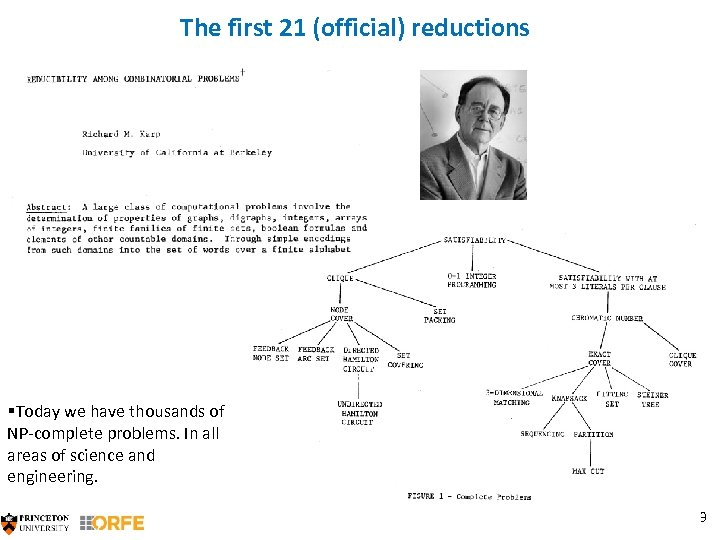The first 21 (official) reductions §Today we have thousands of NP-complete problems. In all areas of science and engineering. 39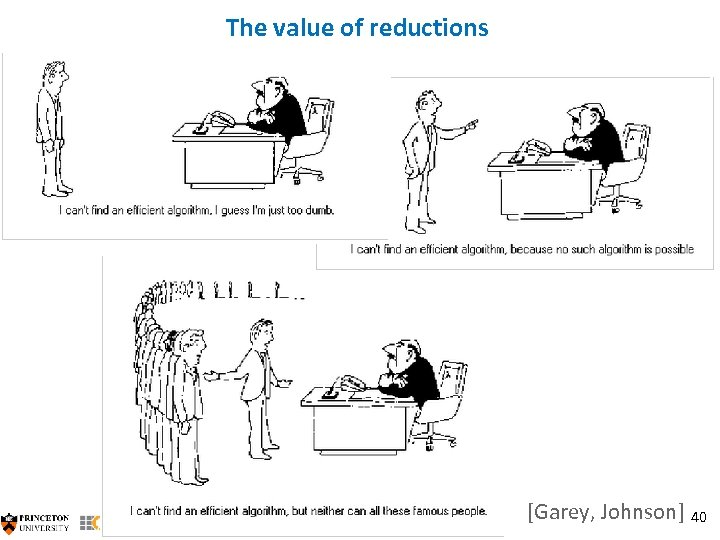The value of reductions [Garey, Johnson] 40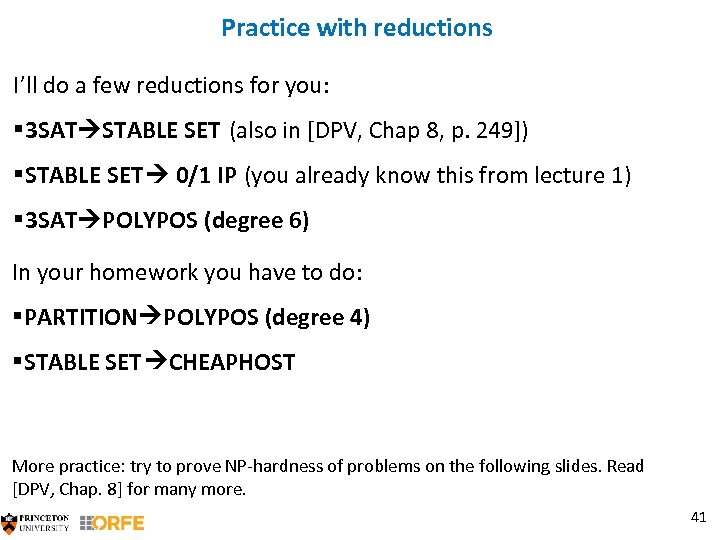Practice with reductions I’ll do a few reductions for you: § 3 SAT STABLE SET (also in [DPV, Chap 8, p. 249]) §STABLE SET 0/1 IP (you already know this from lecture 1) § 3 SAT POLYPOS (degree 6) In your homework you have to do: §PARTITION POLYPOS (degree 4) §STABLE SET CHEAPHOST More practice: try to prove NP-hardness of problems on the following slides. Read [DPV, Chap. 8] for many more. 41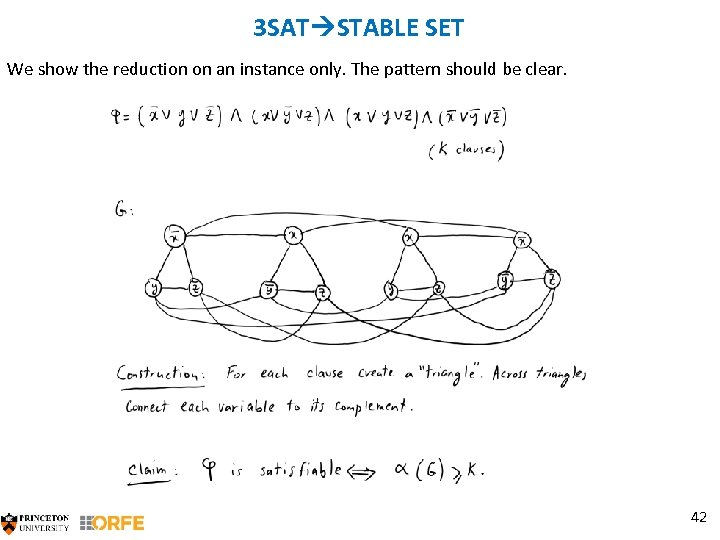3 SAT STABLE SET We show the reduction on an instance only. The pattern should be clear. 42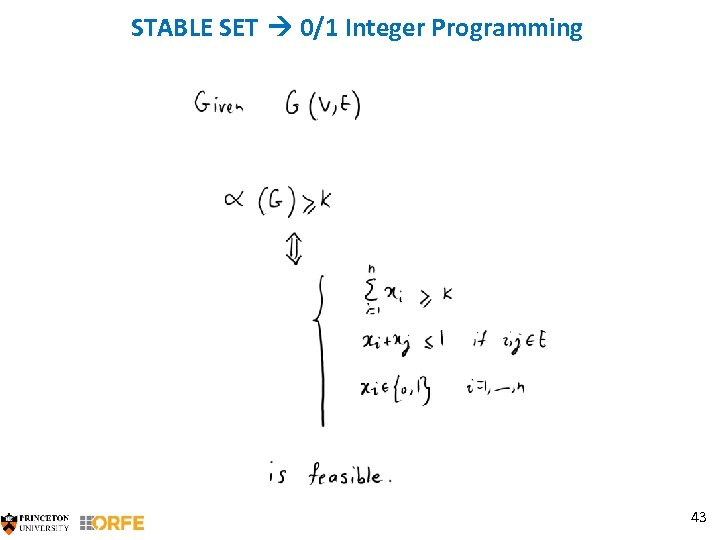STABLE SET 0/1 Integer Programming 43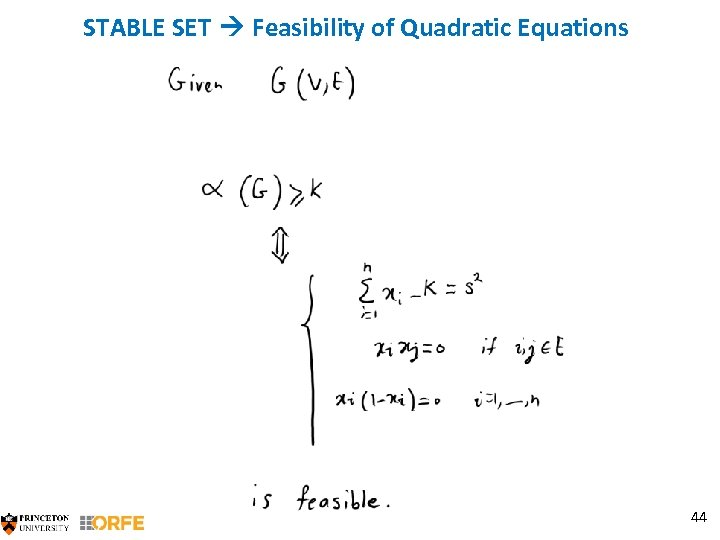STABLE SET Feasibility of Quadratic Equations 44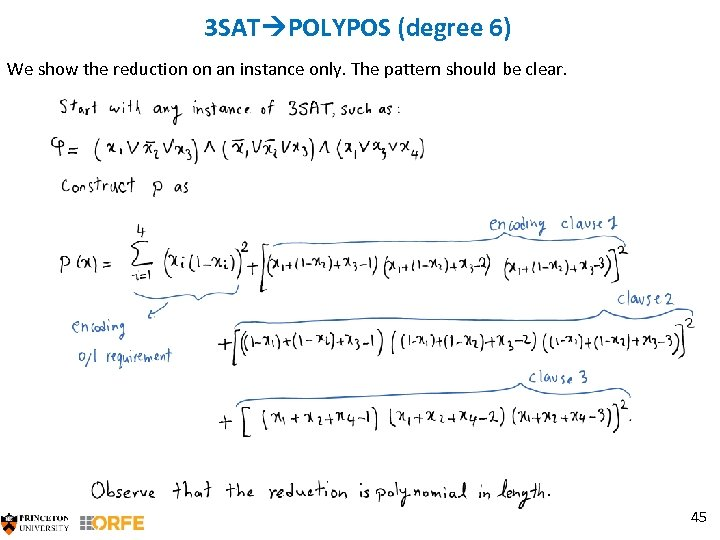3 SAT POLYPOS (degree 6) We show the reduction on an instance only. The pattern should be clear. 45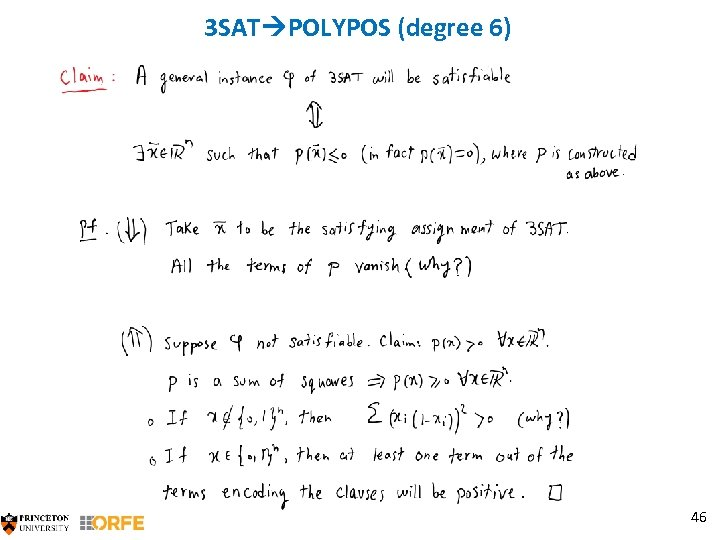3 SAT POLYPOS (degree 6) 46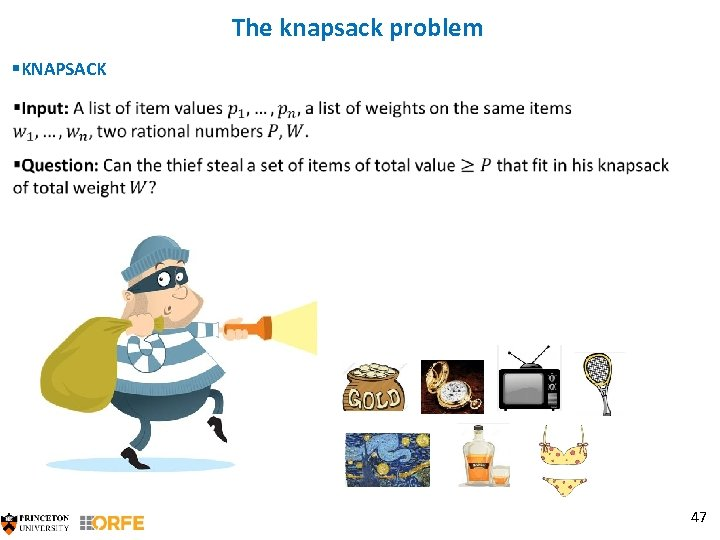The knapsack problem §KNAPSACK 47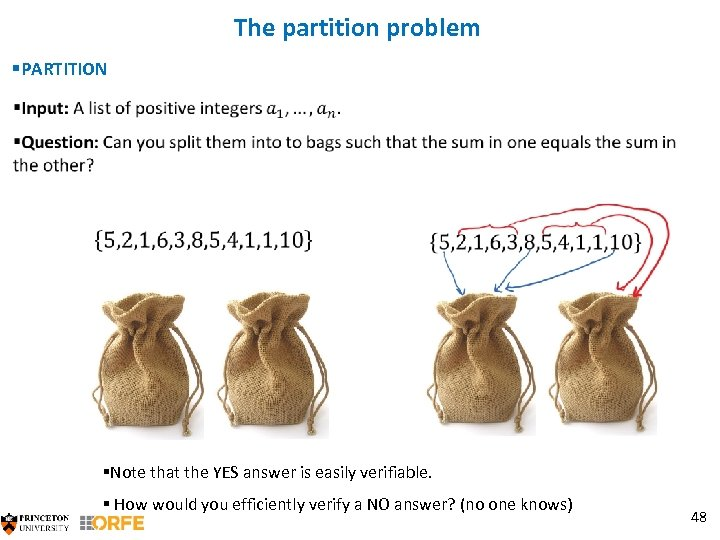The partition problem §PARTITION §Note that the YES answer is easily verifiable. § How would you efficiently verify a NO answer? (no one knows) 48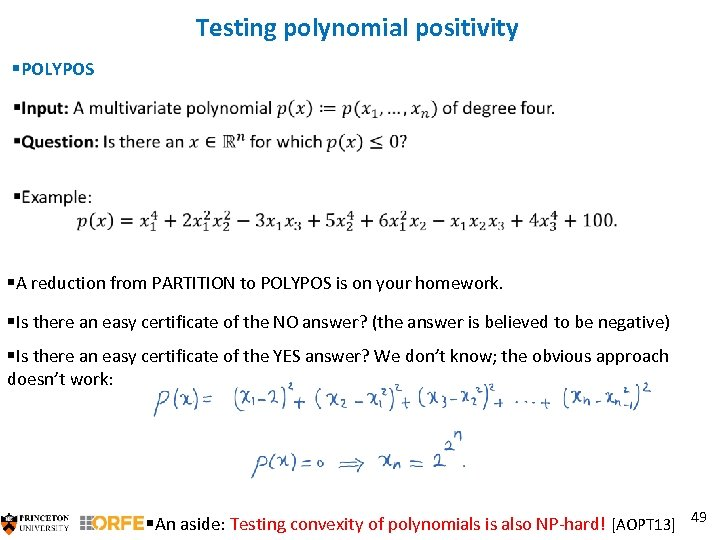Testing polynomial positivity §POLYPOS §A reduction from PARTITION to POLYPOS is on your homework. §Is there an easy certificate of the NO answer? (the answer is believed to be negative) §Is there an easy certificate of the YES answer? We don’t know; the obvious approach doesn’t work: §An aside: Testing convexity of polynomials is also NP-hard! [AOPT 13] 49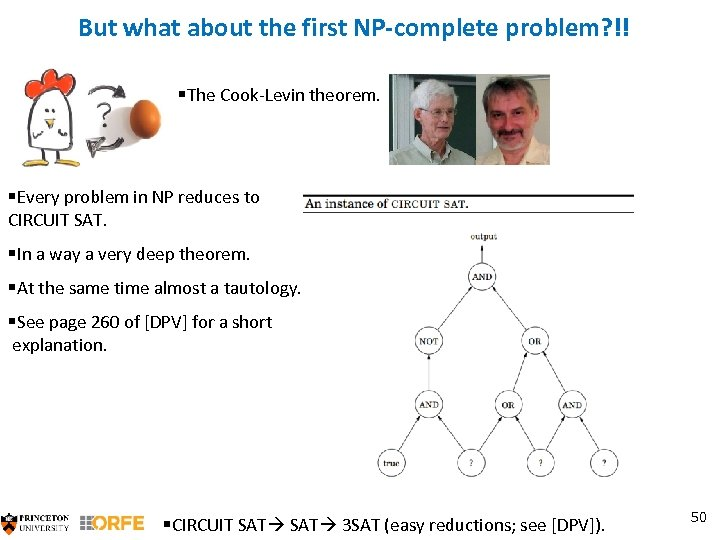But what about the first NP-complete problem? !! §The Cook-Levin theorem. §Every problem in NP reduces to CIRCUIT SAT. §In a way a very deep theorem. §At the same time almost a tautology. §See page 260 of [DPV] for a short explanation. §CIRCUIT SAT 3 SAT (easy reductions; see [DPV]). 50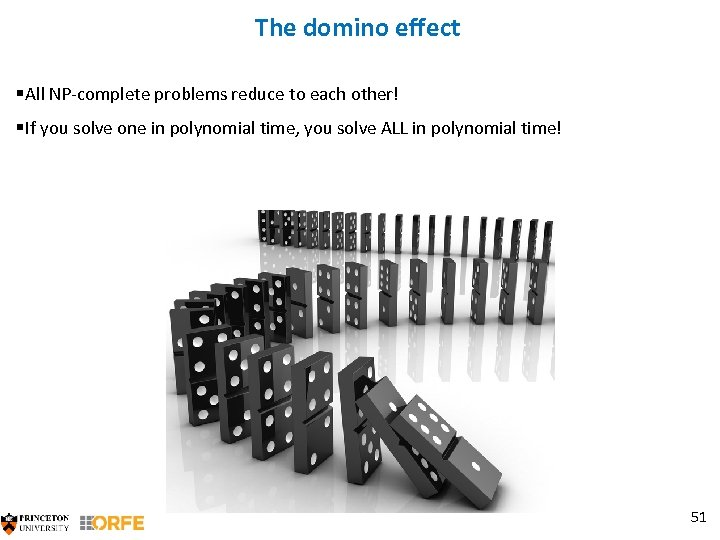The domino effect §All NP-complete problems reduce to each other! §If you solve one in polynomial time, you solve ALL in polynomial time! 51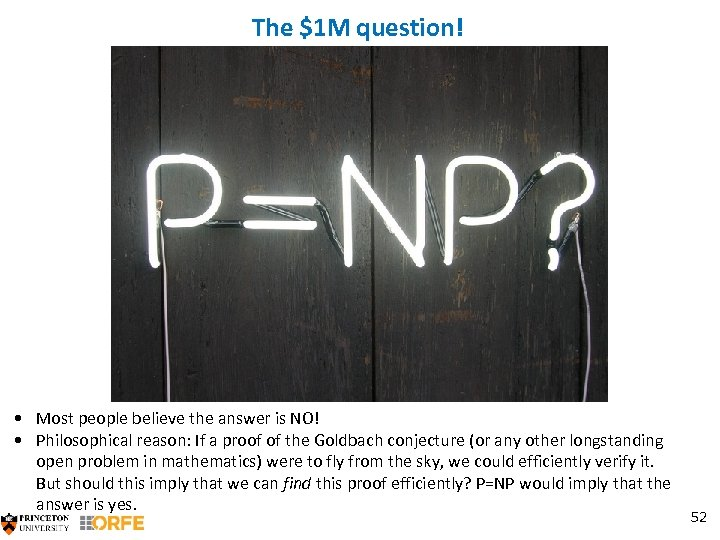The \$1 M question! • Most people believe the answer is NO! • Philosophical reason: If a proof of the Goldbach conjecture (or any other longstanding open problem in mathematics) were to fly from the sky, we could efficiently verify it. But should this imply that we can find this proof efficiently? P=NP would imply that the answer is yes. 52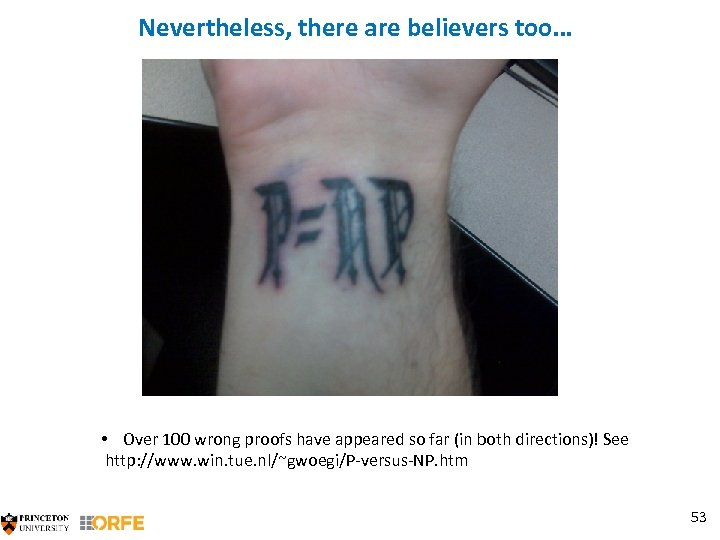Nevertheless, there are believers too… • Over 100 wrong proofs have appeared so far (in both directions)! See http: //www. win. tue. nl/~gwoegi/P-versus-NP. htm 53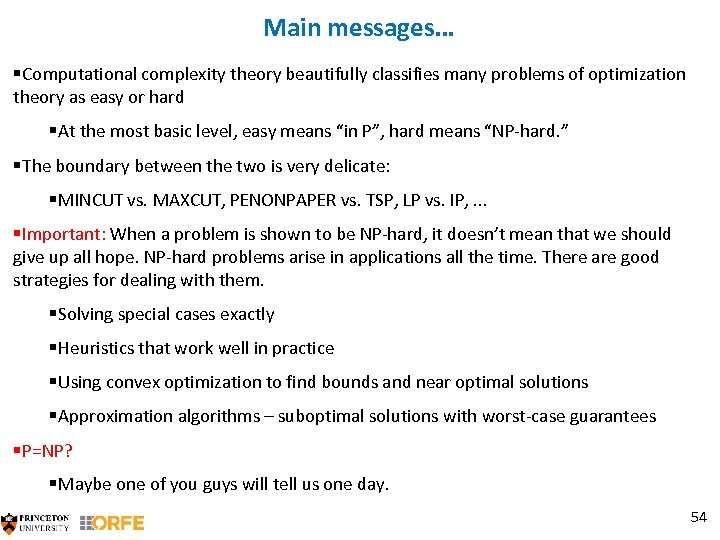Main messages… §Computational complexity theory beautifully classifies many problems of optimization theory as easy or hard §At the most basic level, easy means “in P”, hard means “NP-hard. ” §The boundary between the two is very delicate: §MINCUT vs. MAXCUT, PENONPAPER vs. TSP, LP vs. IP, . . . §Important: When a problem is shown to be NP-hard, it doesn’t mean that we should give up all hope. NP-hard problems arise in applications all the time. There are good strategies for dealing with them. §Solving special cases exactly §Heuristics that work well in practice §Using convex optimization to find bounds and near optimal solutions §Approximation algorithms – suboptimal solutions with worst-case guarantees §P=NP? §Maybe one of you guys will tell us one day. 54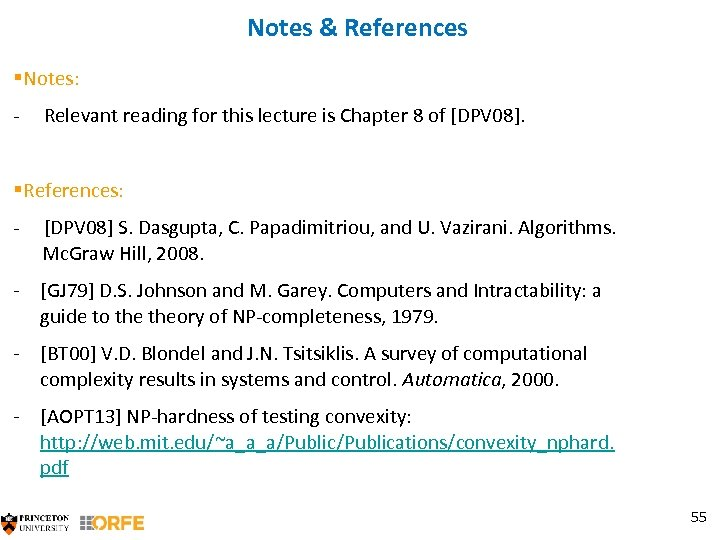Notes & References §Notes: - Relevant reading for this lecture is Chapter 8 of [DPV 08]. §References: - [DPV 08] S. Dasgupta, C. Papadimitriou, and U. Vazirani. Algorithms. Mc. Graw Hill, 2008. - [GJ 79] D. S. Johnson and M. Garey. Computers and Intractability: a guide to theory of NP-completeness, 1979. - [BT 00] V. D. Blondel and J. N. Tsitsiklis. A survey of computational complexity results in systems and control. Automatica, 2000. - [AOPT 13] NP-hardness of testing convexity: http: //web. mit. edu/~a_a_a/Publications/convexity_nphard. pdf 55